# JP2009284684A - Vector controller - Google Patents

## Info

Publication number
JP2009284684A
JP2009284684A JP2008134903A JP2008134903A JP2009284684A JP 2009284684 A JP2009284684 A JP 2009284684A JP 2008134903 A JP2008134903 A JP 2008134903A JP 2008134903 A JP2008134903 A JP 2008134903A JP 2009284684 A JP2009284684 A JP 2009284684A
Authority
JP
Japan
Prior art keywords
value
current
command value
vector control
voltage
Prior art date
Legal status (The legal status is an assumption and is not a legal conclusion. Google has not performed a legal analysis and makes no representation as to the accuracy of the status listed.)
Granted
Application number
JP2008134903A
Other languages
Japanese (ja)
Other versions
JP5150366B2 (en
Inventor
Yoshitaka Iwaji
Kentaro Oi
Kazuaki Tobari

Original Assignee
Hitachi Industrial Equipment Systems Co Ltd

Priority date (The priority date is an assumption and is not a legal conclusion. Google has not performed a legal analysis and makes no representation as to the accuracy of the date listed.)
Filing date
Publication date
Application filed by Hitachi Industrial Equipment Systems Co Ltd, 株式会社日立産機システム filed Critical Hitachi Industrial Equipment Systems Co Ltd
Priority to JP2008134903A priority Critical patent/JP5150366B2/en
Publication of JP2009284684A publication Critical patent/JP2009284684A/en
Application granted granted Critical
Publication of JP5150366B2 publication Critical patent/JP5150366B2/en
Active legal-status Critical Current
Anticipated expiration legal-status Critical

• 244000171263 Ribes grossularia Species 0.000 claims abstract description 240
• 230000001360 synchronised Effects 0.000 claims abstract description 59
• 230000000875 corresponding Effects 0.000 claims description 6
• 230000001276 controlling effects Effects 0.000 claims description 2
• 230000000694 effects Effects 0.000 description 12
• 238000010586 diagrams Methods 0.000 description 9
• 238000000034 methods Methods 0.000 description 4
• 238000006243 chemical reactions Methods 0.000 description 3
• 238000007796 conventional methods Methods 0.000 description 3
• 230000035939 shock Effects 0.000 description 2
• 239000000470 constituents Substances 0.000 description 1
• 230000004907 flux Effects 0.000 description 1
• 230000000051 modifying Effects 0.000 description 1

## Images

•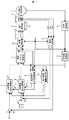•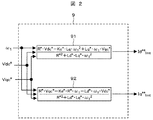•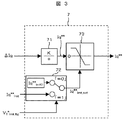•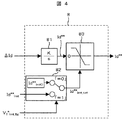•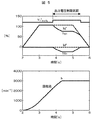••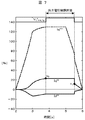•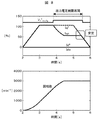•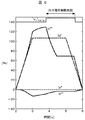••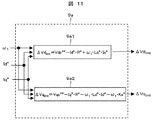•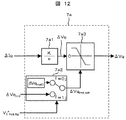•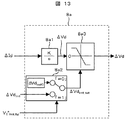•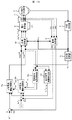•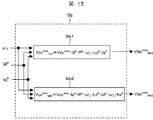•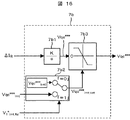•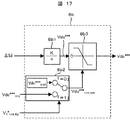•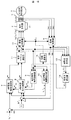•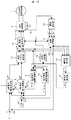•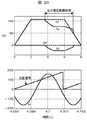•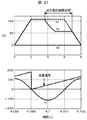## Abstract

<P>PROBLEM TO BE SOLVED: To achieve a high precision/high response motor torque even when an output voltage value of a power converter is limited (saturated) regarding a vector control method of a permanent magnet synchronous motor, and also to provide "a vector controller of the permanent magnet synchronous motor" which can be applied commonly even in a system which performs inexpensive current detection or in a system from which a position detector is omitted. <P>SOLUTION: When the output voltage value of the power converter is limited, an output value of feedback control is calculated, and the output voltage value is limited by using the output voltage value of the converter, a permanent magnet synchronous motor constant (set value), and a frequency calculation value. <P>COPYRIGHT: (C)2010,JPO&INPIT

## Description

The present invention relates to a vector control system of a permanent magnet synchronous motor, and relates to a technique for realizing highly accurate and highly responsive torque control.

Patent Document 1 discloses a conventional technique when the output voltage value of a power converter is limited (saturated). Specifically, the update of a voltage command value created by current control calculation is stopped. A technique for resuming the update of the voltage command value created by the current control calculation when the limit is recovered is disclosed.

In Patent Document 2, a first voltage command value based on a feedforward current control calculation created from a current command value using a constant of an electric motor, and a second voltage command value based on a feedback current control calculation based on a current detection value and a current command value. The output voltage value of the power converter is controlled on the basis of the voltage command value that is an addition value of and the feedback gain of the current control calculation that is the second voltage command value when the output voltage value is limited. A control technique for zeroing is disclosed.

JP 9-28099 A JP 9-47069 A

In the conventional method, when the output voltage value of the power converter is limited (saturated), the load torque or the rotation speed changes greatly, and the output voltage value recovers from the limit, the current control (or the second voltage) (Proportional integral control of command value) is resumed, and at that time, the current value of the motor changes rapidly. This sudden change in the current value causes a problem that a “torque shock” of the motor occurs.

Accordingly, an object of the present invention is to provide a torque with high accuracy and high response even when the load torque or the rotational speed changes greatly from the state where the output voltage value of the power converter is limited and the output voltage value recovers from the limit. An object of the present invention is to provide a “permanent magnet synchronous motor vector control device” capable of realizing control.

When the output voltage value of the power converter is limited, the present invention uses the output voltage value of the converter, the constant (set value) of the permanent magnet synchronous motor, and the frequency calculation value to determine the voltage command value or the current command value. The output voltage value is limited by calculating a limit value of “integral calculation” or “proportional integral calculation” that outputs the signal.

According to the present invention, it is related to the vector control system of a permanent magnet synchronous motor, and even when the output voltage value of the power converter is limited (saturated), highly accurate and highly responsive torque control can be realized. In addition, it is possible to provide a “permanent magnet synchronous motor vector control device” that can be commonly applied to a system that performs inexpensive current detection and a system that omits a position detector.

The present invention is a vector control device that has a power converter that drives a permanent magnet synchronous motor, calculates a voltage command value based on a deviation between a current command value and a current detection value, and controls an output voltage value of the power converter. Thus, when the output voltage value of the power converter is limited, the limit value of the voltage command value is calculated using the output voltage value, the motor constant, the current command value, and the frequency calculation value.

Further, the first voltage command value calculated by the current command value or current detection value, the frequency calculation value and the motor constant is added to the second voltage command value calculated by the deviation between the current command value and the current detection value, The output voltage value of the power converter is controlled, and the limit value of the second voltage command value is calculated using the output voltage value, the motor constant, the current command value, and the frequency calculation value.

Further, a vector control device that controls the output voltage value of the power converter according to the second current command value calculated from the deviation between the first current command value and the detected current value, the frequency calculation value, and the motor constant. Then, when the output voltage value is limited, the limit value of the second current command value is calculated using the output voltage value, the motor constant, and the frequency calculation value.

The present invention also provides a vector control device for controlling an output voltage value of a power converter that drives a permanent magnet synchronous motor, and commands a value of a d-axis current component when the output voltage value of the power converter is limited. When the d-axis current command value is 0, the phase of the zero point of the position detection value θc and the zero point of the u-phase current Iu is the same regardless of whether the output voltage value is limited or not. It is characterized by matching.

Furthermore, in the present invention, in addition to the above-described features, the limit value of the voltage command value, the limit value of the second voltage command value, or the limit value of the second current command value is the output voltage value of the power converter. The calculation is performed using a resistance value, an inductance value, an induced voltage coefficient, and a frequency calculation value that are motor constants.

Further, the polarity of the output value of the voltage command value, the second voltage command value or the second current command value calculated from the deviation between the current command value and the detected current value, and the calculated limit corresponding to each output value When the polarity of the value is different, the calculated limit value is used as the output value.

Further, the output voltage value of the power converter is limited using a limit value of the voltage command value, a limit value of the second voltage command value, or a limit value of the second current command value, and d The output voltage value of the power converter is limited using a limit value of at least one of the axial component and the q-axis component.

Further, according to the present invention, the frequency calculation value is obtained by calculating the deviation between the phase command value and the rotational phase value of the motor from the d-axis and q-axis voltage command values and the detected motor current or the reproduced current. The field of weakness of a permanent magnet motor is characterized in that the current detection value is obtained by reproducing the motor current from the input DC bus current detection value of the power converter. This is a magnetic vector control device.

Hereinafter, embodiments of the present invention will be described in detail with reference to the drawings.

<First embodiment>
FIG. 1 shows an example of the configuration of a “vector control device for a permanent magnet motor” that is an embodiment of the present invention.

1 is a permanent magnet synchronous motor,
2 is a power converter that outputs a voltage proportional to the voltage command values Vu * , Vv * , and Vw * of a three-phase alternating current;
21 is a DC power supply,
3 is a current detector that can detect three-phase alternating currents Iu, Iv, Iw,
4 is a position detector using a resolver or encoder capable of detecting the position θ of the permanent magnet synchronous motor 1;
5 is a frequency calculation unit for calculating a frequency calculation value ω 1 from the position detection value θc;
6 is the detected values Iuc, Ivc, Iwc of the three-phase alternating currents Iu, Iv, Iw,
A coordinate converter that outputs current detection values Idc and Iqc of the d-axis and q-axis from the position detection value θc of the permanent magnet synchronous motor 1;
7 calculates the second q-axis current command value Iq ** according to the deviation between the first q-axis current command value Iq * and the q-axis current detection value Iqc, and uses the output voltage limit flag V 1 * lmt_flg . And
Select limit value Iq ** lmt determined by restriction value Iq ** lmt0 or calculation set internally, q-axis current command calculating unit which outputs the limit Iq **,
8 calculates the second d-axis current command value Id ** according to the deviation between the first d-axis current command value Id * and the detected d-axis current value Idc, and uses the output voltage limit flag V 1 * lmt_flg . And
Select limit value Id ** lmt determined by restriction value Id ** lmt0 or calculation set internally, d-axis current instruction operation section which outputs the limit Id **,
7 and 8 are constituted by integral calculation or proportional calculation + integral calculation.

9 d-axis and q-voltage command value of the axis Vdc *, Vqc * and on the basis of the constant frequency operation value omega 1 and the permanent magnet synchronous motor 1, the limit value of the second current command value Id ** lmt, Iq * * Current command limit calculation unit that outputs lmt ,
10 calculates the output voltage value V 1 * of the power converter from the d-axis and q-axis voltage command values Vdc * and Vdc * ,
When the output voltage command value V 1 * is smaller than the voltage limit value V 1 * max , set the output voltage limit flag V 1 * lmt_flg to “0”,
When V 1 * reaches V 1 * max , the output voltage limit flag V 1 * lmt_flg is set to “1”,
The output voltage limit detection unit is set to

Here, the voltage limit value V 1 * max is a limit value determined from the direct-current voltage value Ed supplied to the power converter. When the power converter is sinusoidally modulated, V 1 * max is (Expression 1). .

In addition, when an in-phase signal (for example, triple harmonic) that is canceled by the line voltage is superimposed on the voltage command values Vu * , Vv * , and Vw * of the three-phase alternating current, V 1 * max is ( Equation 2).

11 is a d-axis voltage command value Vdc based on the electric constant of the permanent magnet synchronous motor 1, the second d-axis current command value Id ** , the second q-axis current command value Iq **, and the frequency command value ω 1. * , A voltage vector calculation unit for calculating the q-axis voltage command value Vqc * ,
A coordinate conversion unit 12 outputs three-phase AC voltage command values Vu * , Vv * , and Vw * from the d-axis and q-axis voltage command values Vdc * and Vdc * and the position detection value θc.

Next, basic operations of voltage control and phase control of the vector control method using the current command limit calculation unit 9 which is a feature of the present invention will be described.

First, regarding the voltage control, the configuration of the current command limit calculation unit 9 will be described with reference to FIG.

91 is expressed by using the d-axis and q-axis voltage command values Vdc * , Vqc * , the frequency calculation value ω 1, and the constants (R * , Ld * , Lq * , Ke * ) of the permanent magnet synchronous motor 1 According to 3), the limit value Id ** lmt of the second d-axis current command value Id ** is calculated.

92, the second q-axis current command value Iq according to (Equation 4) using the d-axis and q-axis voltage command values Vdc * and Vqc * , the frequency calculation value ω 1 and the constant of the permanent magnet synchronous motor 1. calculating a limit value Iq ** lmt of **.

Here, R * is a resistance setting value, Ld * is a d-axis inductance setting value, Lq * is a q-axis inductance setting value, and Ke * is an induced voltage constant setting value.

1 calculates the output voltage value V 1 * using the d-axis and q-axis voltage command values Vdc * and Vqc * by (Equation 5).

Further, using the output voltage command value V 1 * and the voltage limit value V 1 * max , an output voltage limit flag V 1 * lmt_flg is created according to ( Equation 6).

If the output voltage value V 1 of the power converter 2 is limited, these second d-axis current command value limit value Id ** lmt, limit value of the second q-axis current command value Iq ** lmt And the output voltage limit flag V 1 * lmt_flg are used to limit the output values of the q-axis current command calculation unit 7 and the d-axis current command calculation unit 8.

FIG. 3 shows the configuration of the q-axis current command calculation unit 7. Here, an example of an integral operation with a simple configuration is taken as an example.

ΔIq, which is a deviation between the first q-axis current command value Iq * and the q-axis current detection value Iqc, is input to the integration calculation unit 71 whose integral gain is K, and the second q-axis current command value Iq ** is Calculate.

In the limit value selection unit 72, when the output voltage limit flag V 1 * lmt_flg is “0”, the limit value Iq ** lmt0 set in 72 is selected, and when V 1 * lmt_flg is “1” The limit value Iq ** lmt of the second q-axis current command value, which is the output of the current command limit calculation unit 9, is selected and output as the limit value Iq ** lmt_set .

Although the second q-axis current command value Iq ** and the limit value Iq ** lmt_set are input to the limiter 73 , Iq ** and Iq ** lmt_set have the same sign (both positive or negative polarity) for the absolute value of Iq ** | Iq ** | and Iq ** absolute value of lmt_set | Iq ** lmt_set | compare, according equation (7), and outputs the Iq **.

Further, Iq ** and Iq ** lmt_set is different signs (either positive, one negative polarity) in the case of, in accordance with equation (8), and outputs the Iq ** lmt_set as Iq **.

Similarly, in the d-axis current command calculation unit 8 shown in FIG. 4, ΔId which is a deviation between the first d-axis current command value Id * and the d-axis current detection value Idc is an integration calculation unit 81 having an integral gain of K. To calculate the second d-axis current command value Id ** .

In selector 82 of the limit value, when the output voltage limiting flag V 1 * lmt_flg is "0", the 82 limit Id ** lmt0 internally set is, when V 1 * lmt_flg is "1", the current The limit value Id ** lmt of the second d-axis current command value, which is the output of the command limit calculation unit 9, is selected and output as the limit value Id ** lmt_set .

The limit unit 83 receives the second d-axis current command value Id ** and the limit value Id ** lmt_set , and Id ** and Id ** lmt_set have the same sign (both positive or negative polarity). for the absolute value of the Id ** | Id ** | and Id ** absolute value of lmt_set | Id ** lmt_set | compare, according equation (9), and outputs the Id **.

Also, Id ** and Id ** lmt_set is different signs (either positive, one negative polarity) in the case of, in accordance with equation (10), and outputs the Id ** lmt_set as Id **.

Further, in the voltage vector calculation unit 11, the current command values Id ** and Iq ** of the second d axis and the q axis, the frequency calculation value ω 1, and the permanent magnet synchronous motor 1 The constants are used to calculate voltage command values Vdc * and Vqc * for the d-axis and q-axis, and control the output voltage value V 1 of the converter.

On the other hand, in the phase control, the position detector 4 such as a resolver or an encoder detects the position θ of the permanent magnet synchronous motor 1 to obtain a position detection value θc.

The frequency calculation unit 5 uses this position detection value θc to obtain the frequency calculation command value ω 1 by (Equation 12).

The above is the basic operation of voltage control and phase control.

Next, the effect which this invention brings about is demonstrated by a present Example.

In the control device of FIG. 1, the second d-axis current command value limit value Id * of the current command limit calculation unit 9 is used to limit the second d-axis and q-axis current command values Id ** and Iq ** . * lmt, the control characteristics in the case of not using the limit value Iq ** lmt second q-axis current command value will be described.

In the q-axis current command calculation unit 7 and the d-axis current command calculation unit 8, when the output voltage value is not limited (V 1 * lmt_flg = 0), the limit value Iq ** set inside 72 and 82 The outputs of the integral calculation units 71 and 81 are limited by lmt0 and Id ** lmt0 .

However, when the output voltage value is limited (V 1 * lmt_flg = 1), the updating of the integral calculation units 71 and 81 is stopped and the integral calculation is performed when recovery from the limit (V 1 * lmt_flg = 0). The updating of the units 71 and 81 is resumed.

On the other hand, in order to make it equivalent to the method described in Japanese Patent Laid-Open No. 9-47069 which is a conventional method, when “feed forward compensation” is performed in which the first current command value is added to the second current command value, The current command values Id *** and Iq *** are expressed as (Equation 13).

Using this conventional control method, a trapezoidal first q-axis current command value Iq * is given, and the drive control characteristics are shown in FIGS.

FIG. 5 shows control characteristics when the constants of the permanent magnet synchronous motor 1 and the constants set in the voltage vector calculation unit 11 match. It can be seen that the vehicle has accelerated from point a and reached the maximum number of revolutions at point b. Here, in the section where the output voltage value V 1 of the power converter is limited (V 1 * lmt_flg = 1), the d-axis and q-axis current detection values Idc and Iqc are the first d-axis current command values Id. * Although it does not follow the first q-axis current command value Iq * , it can be seen that the control operation is stable.

On the other hand, FIG. 6 shows the control when the constant of the permanent magnet synchronous motor 1 does not match the constant set in the voltage vector calculation unit 11 (Lq * = 0.8 × Lq, Ld * = 0.8 × Ld). It is a characteristic.

In this case, if there is a setting error in the voltage vector calculation unit 11, an “overshoot” occurs in the q-axis current detection value Iqc in the region A of FIG. 6, and the motor torque is caused by this. “Shock” occurs.

FIG. 7 shows waveforms of the second d-axis and q-axis current command values Id ** and Iq ** used in (Equation 13).

In the section where the output voltage value is limited (V 1 * lmt_flg = 1), the output values Id ** and Iq ** of the integration calculation unit are held from the point c 0 and c recovered from the limit. It can be seen that at one point, Id ** and Iq ** change rapidly.

This is the “cause of overshoot” of the q-axis current detection value Iqc.

In other words, depending on the rotational speed of the permanent magnet synchronous motor 1 and the condition of the torque command value to be applied, the optimum value of Iq ** differs between the c 0 point immediately before entering the output voltage limit section and the c 1 point recovered from the limit. I understand.

Therefore, when the output voltage value V 1 is limited, instead of a method of holding the value of the integral calculation unit, a new current command limit calculation unit 9 is provided, and continuously depending on the conditions of the rotational speed and the torque command value to be given. Limit the output of the integral calculation unit.

The current command limit calculation unit 9 that is a feature of the present invention uses the d-axis and q-axis voltage command values Vdc * and Vqc * , the frequency calculation value ω 1, and the constants of the permanent magnet synchronous motor 1 to determine the rotation frequency and current. Depending on the state of the command value, the limit values Id ** lmt and Iq ** lmt of the second d-axis and q-axis current command values Id ** and Iq ** are calculated.

FIG. 8 shows the characteristics (Lq * = 0.8 × Lq, Ld * = 0.8 × Ld) when the output voltage saturation control (using FIGS. 7 and 8) according to the present invention is performed.

Even when the constants of the permanent magnet synchronous motor 1 and the constants set in the voltage vector calculation unit 11 do not match, there is no overshoot of Iqc in the A region as in the prior art (FIG. 6), and even when recovering from the limitation. It turns out that it becomes a stable control characteristic.

FIG. 9 shows waveforms of the second d-axis and q-axis current command values Id ** and Iq ** , which are output values of the integral calculation unit in the q-axis current command calculation unit 7 and the d-axis current command calculation unit 8. Show.

Even in a section where the output voltage value V 1 is limited (V 1 * lmt_flg = 1) , Id **, Iq ** is it can be seen being continuously limited.

In this embodiment, the calculation configuration of the q-axis current command calculation unit 7 and the d-axis current command calculation unit 8 is an integral calculation, but the same effect as in this embodiment can be obtained even when the proportional calculation + integral calculation is used. Can do.

<Second Embodiment>
FIG. 10 shows a second embodiment of the present invention.

The present embodiment is an example in which the present invention is applied to a vector control device provided with d-axis and q-axis current control arithmetic units. In the figure, components 1 to 6, 10, 12, and 21 are the same as those in FIG.

7a calculates a second q-axis voltage command value ΔVq in accordance with the deviation between the first q-axis current command value Iq * and the q-axis current detection value Iqc, and from the output voltage limit flag V 1 * lmt_flg , the set limit value ΔVq lmt0, or select the limit value? Vq lmt of the second q-axis voltage command value calculated by the calculation, q-axis current control calculation section for outputting the limit? Vq, 8a the first d-axis depending on the deviation between the current command value Id * and the d-axis current detection value Idc, the second d-axis voltage command value .DELTA.Vd calculated from the output voltage limiting flag V 1 * lmt_flg, limit .DELTA.Vd Lmt0 set internally, Alternatively, a d-axis current control calculation unit that selects and outputs a limit value ΔVd lmt of the second d-axis voltage command value obtained by calculation and limits ΔVd is represented.

Further, 9a voltage command value Vdc ** of the d-axis and q-axis, Vqc ** and frequency calculation value omega 1 and the first d-axis and the current command value of q-axis Id *, Iq * and the permanent magnet synchronous motor 1 Is a voltage command limit calculation unit that outputs a limit value ΔVd lmt of the second d-axis voltage command value and a limit value ΔVd lmt of the second d-axis voltage command value, and 11 a is a permanent magnet synchronous motor 1. Based on the constant, the first d-axis and q-axis current command values Id * and Iq * , the frequency command value ω 1 and the current control output values ΔVd and ΔVq, the d-axis and q-axis voltage command values Vdc ** and Vqc Represents a voltage vector calculation unit for calculating ** .

Next, the configuration of the voltage command limit calculation unit 9a will be described with reference to FIG.

9a1 is a d-axis voltage command value Vdc ** , a frequency calculation value ω 1 , first d-axis and q-axis current command values Id * , Iq *, and constants (R * , Ld *) of the permanent magnet synchronous motor 1 . , Lq * , Ke * ), the limit value ΔVd lmt of the second d-axis voltage command value ΔVd is calculated according to ( Equation 14).

9 a 2, the q-axis voltage command value Vqc ** , the frequency calculation value ω 1 , the first d-axis and q-axis current command values Id * , Iq *, and the constants (R * , Ld *) of the permanent magnet synchronous motor 1 . , Lq * , Ke * ), the limit value ΔVq lmt of the second q-axis voltage command value ΔVq is calculated according to ( Equation 15).

FIG. 12 shows the configuration of the q-axis current control calculation unit 7a. Here, an example of an integral operation with a simple configuration is taken as an example.

ΔIq, which is a deviation between the first q-axis current command value Iq * and the q-axis current detection value Iqc, is input to the integral calculation unit 7a1 having an integral gain K, and calculates the second q-axis voltage command value ΔVq. . In selecting unit 7a2 limits, when the output voltage limiting flag V 1 * lmt_flg is "0", the limitation value? Vq Lmt0 internally set 7a2, when V 1 * lmt_flg is "1", the voltage command limit The limit value ΔVq lmt of the second q-axis voltage command value, which is the output of the calculation unit 9a, is selected and output as the limit value ΔVq lmt_set .

The limit unit 7a3 receives the second q-axis voltage command value ΔVq and the limit value ΔVq lmt_set . If ΔVq and ΔVq lmt_set have the same sign (both positive or negative polarity), the absolute value of ΔVq |? Vq | and? Vq absolute value of lmt_set | ΔVq lmt_set | compare, according equation (16), and outputs the? Vq.

In addition, when ΔVq and ΔVq lmt_set have different signs (one is positive and one is negative)
According to ( Equation 17), ΔVq lmt_set is output as ΔVq.

FIG. 13 shows the configuration of the d-axis current control calculation unit 8a. Here too, an example of an integral operation with a simple configuration is taken.

ΔId, which is the deviation between the first d-axis current command value Id * and the detected d-axis current value Idc, is input to the integral calculation unit 8a1 whose integral gain is K, and calculates the second d-axis voltage command value ΔVd. . In the limit value selection unit 8a2, when the output voltage limit flag V 1 * lmt_flg is “0”, the limit value ΔVd lmt0 set inside 8a2 is selected, and the output voltage limit flag V 1 * lmt_flg is “1”. At this time, the limit value ΔVd lmt of the second d-axis voltage command value, which is the output of the voltage command limit calculation unit 9a, is selected and output as the limit value ΔVd lmt_set .

The limit unit 8a3 receives the second d-axis voltage command value ΔVd and the limit value ΔVd lmt_set . If ΔVd and ΔVd lmt_sett have the same sign (both positive or negative polarity), the absolute value of ΔVd | .DELTA.Vd | and .DELTA.Vd absolute value of lmt_set | ΔVd lmt_set | compare, according equation (18), and outputs the .DELTA.Vd.

If ΔVd and ΔVd lmt_set have different signs (one is positive and one is negative)
According to ( Equation 19), ΔVq lmt_set is output as ΔVq.

Further, in the voltage vector calculation unit 11a, as shown in (Equation 20), the first d-axis and q-axis current command values Id * and Iq * , the frequency calculation value ω 1 and the constants of the permanent magnet synchronous motor 1 are set. Are used to calculate the first d-axis and q-axis voltage command values Vdc * and Vqc * , add the second d-axis and q-axis voltage command values ΔVd and ΔVq, and output the converter output voltage value. to control the V 1.

Even in this embodiment, the same effect as that of the first embodiment can be obtained.

Also in this embodiment, the calculation configuration of the q-axis current control calculation unit 7a and the d-axis current control calculation unit 8a is an integral calculation, but the same effect as in this embodiment can be obtained even if the proportional + integral calculation is performed. it can.

<Third embodiment>
FIG. 14 shows a third embodiment of the present invention.

The present embodiment is an example in which the present invention is applied to a vector control device provided with d-axis and q-axis current control arithmetic units (feedback control only). In the figure, components 1 to 6, 10, 12, and 21 are the same as those in FIG.

7b calculates a q-axis voltage command value Vqc *** in accordance with the deviation between the first q-axis current command value Iq * and the q-axis current detection value Iqc, and from the output voltage limit flag V 1 * lmt_flg , the set limit value Vqc *** lmt0, or select the limit value Vqc *** lmt of q-axis voltage command value calculated by the calculation, q-axis current control calculation section for outputting the limit Vqc ***, 8b Calculates the d-axis voltage command value Vdc *** according to the deviation between the first d-axis current command value Id * and the detected d-axis current value Idc, and internally sets it from the output voltage limit flag V 1 * lmt_flg the limit value Vdc *** lmt0, or select the limit value Vdc *** lmt of d-axis voltage command value calculated by the calculation represents the d-axis current control calculation section for outputting the limit Vdc ***.

Reference numeral 9b denotes d-axis and q-axis voltage command values Vdc *** and Vqc *** , a frequency calculation value ω 1 , first d-axis and q-axis current command values Id * and Iq *, and permanent magnet synchronization. Based on the constants of the electric motor 1, a voltage command limit calculation unit that outputs limit values Vdc *** lmt and Vqc *** lmt for the d-axis and q-axis voltage command values is represented.

The configuration of the voltage command limit calculation unit 9b will be described with reference to FIG.

9b1 is the voltage command value of d-axis Vdc *** and frequency calculation value omega 1 and a current command value of the first d-axis and q-axis Id *, Iq * and the permanent magnet synchronous motor 1 constant (R *, Ld * , Lq * , Ke * ) is used to calculate the limit value Vdc *** lmt of the d-axis voltage command value Vdc *** according to ( Equation 21).

In 9b2, voltage command value of the q-axis Vqc *** and the current command value of the frequency calculation value omega 1 and the first d-axis and q-axis Id *, Iq * and the permanent magnet synchronous motor 1 constant (R *, Ld * , Lq * , Ke * ) and the limit value Vqc *** lmt of the q-axis voltage command value Vqc *** is calculated according to ( Equation 22).

FIG. 16 shows the configuration of the q-axis current control calculation unit 7b. Here, an example of an integral operation with a simple configuration is taken as an example.

ΔIq, which is a deviation between the first q-axis current command value Iq * and the q-axis current detection value Iqc, is input to the integral calculation unit 7b1 whose integral gain is K, and calculates the q-axis voltage command value Vqc *** . .

In the limit value selection unit 7b2 , when the output voltage limit flag V 1 * lmt_flg is “0”, the limit value Vqc *** lmt0 set in 7b2 is set, and the output voltage limit flag V 1 * lmt_flg is “1”. In this case, the limit value Vqc *** lmt of the q-axis voltage command value, which is the output of the voltage command limit calculation unit 9b, is selected and output as the limit value Vqc *** _ set.

The q-axis voltage command value Vqc *** and the limit value Vqc *** lmt_set are input to the limiter 7b3, but Vqc *** and Vqc *** lmt_sett have the same sign (both positive or negative polarity) case of) the absolute value of Vqc *** | Vqc *** | and Vqc *** absolute value of lmt_set | Vqc *** lmt_set | compare, according equation (23), outputs Vqc *** To do.

Further, Vqc *** and Vqc *** lmt_set is different signs (either positive, one negative polarity) in the case of, in accordance with equation (24), and outputs the Vqc *** lmt_set as Vqc *** .

FIG. 17 shows the configuration of the d-axis current control calculation unit 8b.

ΔId, which is a deviation between the first d-axis current command value Id * and the detected d-axis current value Idc, is input to the integral calculation unit 8b1 whose integral gain is K, and calculates the d-axis voltage command value Vdc *** . .

In the limit value selection unit 8b2, when the output voltage limit flag V 1 * lmt_flg is “0”, the limit value Vdc *** lmt0 set in 78b2 is set, and the output voltage limit flag V 1 * lmt_flg is “1”. In this case, the limit value Vdc *** lmt of the d-axis voltage command value, which is the output of the voltage command limit calculation unit 9b, is selected and output as the limit value Vdc *** lmt_set .

The d-axis voltage command value Vdc *** and the limit value Vdc *** lmt_set are input to the limiter 8b3, but Vdc *** and Vdc *** lmt_set have the same sign (both positive or negative polarity) case of) the absolute value of Vdc *** | Vdc *** | and Vdc *** absolute value of lmt_set | Vdc *** lmt_set | compare, according equation (25), outputs a Vdc *** To do.

Further, Vdc *** and Vdc *** lmt_set is different signs (either positive, one negative polarity) in the case of, in accordance with equation (26), and outputs a Vdc *** lmt_set as Vdc *** .

Even in this embodiment, the same effect as that of the first embodiment can be obtained.

In the present embodiment, the calculation configuration of the q-axis current control calculation unit 7b and the d-axis current control calculation unit 8b is an integral calculation. However, the same effect as in the present embodiment can be obtained even when the proportional + integral calculation is performed. it can.

<Fourth embodiment>
FIG. 18 shows a fourth embodiment of the present invention.

This embodiment is applied to a control device in which a position detector such as a resolver or an encoder is omitted. In the figure, components 1-3, 6-12, and 21 are the same as those in FIG.

13 is based on the d-axis and q-axis voltage command values Vdc * , Vqc * , the d-axis and q-axis current detection values Idc, Iqc, the frequency calculation value ω 1, and the constant of the permanent magnet synchronous motor 1 (Equation 27) 2 represents an error calculation unit that estimates a phase error Δθc (= θc′−θ) that is a deviation between the phase command value θc ′ and the motor phase value θ.

14 is a frequency estimator for calculating the estimated frequency value ω 1 ′ so that the phase error Δθc is “zero”, and 15 is a phase for calculating the phase command value θc by integrating the estimated frequency value ω 1 ′. Represents an arithmetic unit.

Even in such an inexpensive position sensorless control system, it is obvious that the operation is the same as in the above-described embodiment and the same effect can be obtained.

In this embodiment, the calculation configuration of the q-axis current control calculation unit 7 and the d-axis current control calculation unit 8 is an integral calculation. However, the same effect as in this embodiment can be obtained even when the proportional + integral calculation is performed. it can.

Also, the same effects as in this embodiment can be obtained by using the voltage vector calculation and the current control calculation in the second and third embodiments.

<Fifth embodiment>
Up to the above first to fourth embodiments, the three-phase AC currents Iu to Iw detected by the expensive current detector 3 have been detected. However, the present invention is a control that performs inexpensive current detection. The present invention can also be applied to an apparatus.

FIG. 19 shows a fifth embodiment. In the figure, constituent elements 1, 2, 6 to 15, 21 are the same as those in FIG.

16 is a current estimator that estimates a DC current I DC flowing through the input bus of the power converter, the AC currents Iu 3 phase flowing to the permanent magnet synchronous motor 1, Iv, and Iw. Using the estimated current values Iu ^, Iv ^, Iw ^, the coordinate conversion unit 6 calculates current detection values Idc, Iqc for the d-axis and the q-axis.

Even in such an inexpensive current sensorless control system, it is obvious that the operation is the same as in the embodiment and the same effect can be obtained.

In this embodiment, the calculation configuration of the q-axis current control calculation unit 7 and the d-axis current control calculation unit 8 is an integral calculation. However, the same effect as in this embodiment can be obtained even when the proportional + integral calculation is performed. it can.

Also, the same effects as in this embodiment can be obtained by using the voltage vector calculation and the current control calculation in the second and third embodiments.

In the embodiment according to the present invention, even when the output voltage value of the power converter is limited, the value of the d-axis (magnetic flux) axis current component can be made to follow the command value. Here, the upper side of FIG. 20 is the conventional control characteristic (corresponding to FIG. 6), and the lower side is “the relationship between the position detection value θc and the u-phase current Iu of the three-phase alternating current in the output voltage limiting section at this time. Is shown. In the conventional method, even if Id * = 0, since Id is generated, the zero point of the position detection value θc and the phase of the zero point of the u-phase current Iu do not match (when Id = 0, they match). .

On the other hand, the upper side of FIG. 21 is the control characteristic of the present invention (corresponding to FIG. 8), and the lower side is “the relationship between the position detection value θc and the u-phase current Iu of the three-phase alternating current in the output voltage limiting section at this time. Is shown.

In the present invention, when Id * = 0, it can be seen that the phase of the zero point of the position detection value θc and the phase of the zero point of the u-phase current Iu match. As described above, regardless of whether or not the output voltage value of the power converter is limited, it is clear from the relationship between the position signal of the permanent magnet synchronous motor 1 or the reference signal of the control shaft and the phase of the motor current.

The block diagram of the vector control apparatus of the permanent-magnet synchronous motor which shows one Example of this invention. Explanatory drawing of the current command limitation calculating part 9 in the control apparatus of FIG. Explanatory drawing of the q-axis current command calculating part 7 in the control apparatus of FIG. Explanatory drawing of the d-axis current command calculating part 8 in the control apparatus of FIG. Fig. 3 is a control characteristic diagram of a conventional control method (when the control constants match). Control characteristic diagram of conventional control method (when control constants do not match). FIG. 7 is a control characteristic diagram of a third d-axis current command value Id *** and a q-axis current command value Iq *** in the case of FIG. 6. The control characteristic figure in a present Example (when a control constant does not correspond). FIG. 9 is a characteristic diagram of the second d-axis current command value Id ** and the q-axis current command value Iq ** in the case of FIG. 8. The block diagram of the vector control apparatus of the permanent-magnet synchronous motor which shows the other Example of this invention. Explanatory drawing of the voltage command limitation calculating part 9a in the control apparatus of FIG. Explanatory drawing of the q-axis current control calculating part 7a in the control apparatus of FIG. Explanatory drawing of the d-axis current control calculating part 8a in the control apparatus of FIG. The block diagram of the vector control apparatus of the permanent-magnet synchronous motor which shows the other Example of this invention. Explanatory drawing of the voltage command limitation calculating part 9b in the control apparatus of FIG. Explanatory drawing of the q-axis current control calculating part 7b in the control apparatus of FIG. Explanatory drawing of the d-axis current control calculating part 8b in the control apparatus of FIG. The block diagram of the vector control apparatus of the permanent-magnet synchronous motor which shows the other Example of this invention. The block diagram of the vector control apparatus of the permanent-magnet synchronous motor which shows the other Example of this invention. The control characteristic figure of the conventional control system. The control characteristic figure concerning a present Example.

Explanation of symbols

DESCRIPTION OF SYMBOLS 1 Permanent magnet synchronous motor 2 Power converter 3 Current detector 4 Position detector 5 Frequency calculating part 6 Coordinate converting part 7 q-axis current command calculating part 7a, 7b q-axis current control calculating part 8 d-axis current command calculating part 8a, 8b d-axis current control calculation unit 9 current command limit calculation units 9a and 9b voltage command limit calculation unit 10 output voltage limit detection unit 11 voltage vector calculation unit 12 coordinate conversion unit 13 axis error calculation unit 14 frequency estimation unit 15 phase calculation unit 16 Current estimation unit 21 DC power supply Id * first d-axis current command value Id ** second d-axis current command value Id *** third d-axis current command value Iq * first q-axis current command value Iq ** Second q-axis current command value Iq *** Third q-axis current command value Idc d-axis current detection value Iqc d-axis current detection value Id ** lmt Second d-axis current command value limit value Iq ** lmt second q-axis current command value limit value V1 * lmt_flg output voltage limit flag ΔVd lmt Second d-axis voltage command value limit value ΔVq lmt Second q-axis voltage command value limit value Vdc *** lmt d-axis voltage command value limit value Vqc *** lmt q-axis voltage command value Limit values Vdc * , Vdc ** , Vdc *** d-axis voltage command value Vqc * , Vqc ** , Vqc *** q-axis voltage command value V1 * Output voltage value θc Position detection value ω 1 Frequency calculation value

## Claims (20)

1. It has a power converter that drives a permanent magnet synchronous motor,
A vector control device that calculates a voltage command value based on a deviation between a current command value and a current detection value, and controls an output voltage value of the power converter,
When the output voltage value of the power converter is limited, the limit value of the voltage command value is calculated using the output voltage value, the motor constant, the current command value, and the frequency calculation value. Vector control device for magnet synchronous motor.
2. It has a power converter that drives a permanent magnet synchronous motor,
Adding a first voltage command value calculated from a current command value or current detection value, a frequency calculation value and a motor constant, and a second voltage command value calculated from a deviation between the current command value and the current detection value; A vector control device for controlling an output voltage value of the power converter,
When the output voltage value of the power converter is limited, the limit value of the second voltage command value is calculated using the output voltage value, the motor constant, the current command value, and the frequency calculation value. A vector control device for a permanent magnet synchronous motor, characterized in that the calculation is performed.
3. It has a power converter that drives a permanent magnet synchronous motor,
A second current command value calculated from a deviation between the first current command value and the detected current value;
A vector control device that controls an output voltage value of the power converter according to a frequency calculation value and a motor constant,
When the output voltage value is limited, the limit value of the second current command value is calculated using the output voltage value, the electric motor constant, and the frequency calculation value. Vector controller for permanent magnet synchronous motor.
4. The vector control device according to claim 1,
The limit value of the voltage command value, the limit value of the second voltage command value, or the limit value of the second current command value includes an output voltage value of the power converter, a resistance value that is the motor constant, A vector control device for a permanent magnet synchronous motor, wherein the vector control device calculates using an inductance value, an induced voltage coefficient, and a frequency calculation value.
5. The vector control device according to claim 1,
A voltage command value calculated by a deviation between the current command value and the current detection value, a polarity of an output value of the second voltage command value or the second current command value,
A vector control device for a permanent magnet synchronous motor, wherein the calculated limit value is used as an output value when the polarity of the calculated limit value corresponding to each output value is different.
6. The vector control device according to claim 1,
The output voltage value of the power converter is limited using the limit value of the voltage command value, the limit value of the second voltage command value, or the limit value of the second current command value. A permanent magnet synchronous motor vector control device.
7. The vector control device according to claim 6, wherein
Using the limit value of at least one of the d-axis component and the q-axis component,
A vector control device for a permanent magnet synchronous motor, wherein the output voltage value of the power converter is limited.
8. The vector control device according to claim 1,
The frequency calculation value is obtained by calculating the deviation between the phase command value and the rotational phase value of the motor from the d-axis and q-axis voltage command values and the detected motor current or reproduced current so that the deviation becomes zero. A vector controller for a permanent magnet motor, characterized in that it is obtained by calculation.
9. The vector control device according to claim 1,
The field-weakening vector control device for a permanent magnet motor, wherein the current detection value is a current that reproduces a motor current from an input DC bus current detection value of the power converter.
10. A vector control device that controls an output voltage value of a power converter that drives a permanent magnet synchronous motor,
When the output voltage value of the power converter is limited, the value of the d-axis current component is made to follow the command value.
11. A vector control device that controls an output voltage value of a power converter that drives a permanent magnet synchronous motor,
Regardless of whether the output voltage value is limited or not, when the d-axis current command value is 0, the phase of the zero point of the position detection value θc and the zero point of the u-phase current Iu are the same. Vector controller for permanent magnet synchronous motor.
12. The vector control device according to claim 2, wherein
The limit value of the voltage command value, the limit value of the second voltage command value, or the limit value of the second current command value includes an output voltage value of the power converter, a resistance value that is the motor constant, A vector control device for a permanent magnet synchronous motor, wherein the vector control device calculates using an inductance value, an induced voltage coefficient, and a frequency calculation value.
13. The vector control device according to claim 2, wherein
A voltage command value calculated by a deviation between the current command value and the current detection value, a polarity of an output value of the second voltage command value or the second current command value,
A vector control device for a permanent magnet synchronous motor, wherein the calculated limit value is used as an output value when the polarity of the calculated limit value corresponding to each output value is different.
14. The vector control device according to claim 2, wherein
The output voltage value of the power converter is limited using the limit value of the voltage command value, the limit value of the second voltage command value, or the limit value of the second current command value. A permanent magnet synchronous motor vector control device.
15. The vector control device according to claim 2, wherein
The frequency calculation value is obtained by calculating the deviation between the phase command value and the rotational phase value of the motor from the d-axis and q-axis voltage command values and the detected motor current or reproduced current so that the deviation becomes zero. A vector controller for a permanent magnet motor, characterized in that it is obtained by calculation.
16. The vector control device according to claim 2, wherein
The field-weakening vector control device for a permanent magnet motor, wherein the current detection value is a current that reproduces a motor current from an input DC bus current detection value of the power converter.
17. The vector control device according to claim 3, wherein
The limit value of the voltage command value, the limit value of the second voltage command value, or the limit value of the second current command value includes an output voltage value of the power converter, a resistance value that is the motor constant, A vector control device for a permanent magnet synchronous motor, wherein the vector control device calculates using an inductance value, an induced voltage coefficient, and a frequency calculation value.
18. The vector control device according to claim 3, wherein
A voltage command value calculated by a deviation between the current command value and the current detection value, a polarity of an output value of the second voltage command value or the second current command value,
A vector control device for a permanent magnet synchronous motor, wherein the calculated limit value is used as an output value when the polarity of the calculated limit value corresponding to each output value is different.
19. The vector control device according to claim 3, wherein
The output voltage value of the power converter is limited using the limit value of the voltage command value, the limit value of the second voltage command value, or the limit value of the second current command value. A permanent magnet synchronous motor vector control device.
20. The vector control device according to claim 3, wherein
The frequency calculation value is obtained by calculating the deviation between the phase command value and the rotational phase value of the motor from the d-axis and q-axis voltage command values and the detected motor current or reproduced current so that the deviation becomes zero. A vector controller for a permanent magnet motor, characterized in that it is obtained by calculation.
JP2008134903A 2008-05-23 2008-05-23 Vector control equipment Active JP5150366B2 (en)

## Priority Applications (1)

Application Number Priority Date Filing Date Title
JP2008134903A JP5150366B2 (en) 2008-05-23 2008-05-23 Vector control equipment

## Applications Claiming Priority (1)

Application Number Priority Date Filing Date Title
JP2008134903A JP5150366B2 (en) 2008-05-23 2008-05-23 Vector control equipment

## Publications (2)

Publication Number Publication Date
JP2009284684A true JP2009284684A (en) 2009-12-03
JP5150366B2 JP5150366B2 (en) 2013-02-20

# Family

## Family Applications (1)

Application Number Title Priority Date Filing Date
JP2008134903A Active JP5150366B2 (en) 2008-05-23 2008-05-23 Vector control equipment

## Country Status (1)

JP (1) JP5150366B2 (en)

## Cited By (3)

* Cited by examiner, † Cited by third party
Publication number Priority date Publication date Assignee Title
JP2012151931A (en) * 2011-01-17 2012-08-09 Nagaoka Univ Of Technology Motor controller
CN103825518A (en) * 2014-03-05 2014-05-28 华侨大学 System and method for phase sequence detection and rotor initial location positioning of three-phase permanent-magnet synchronous motor
WO2015093175A1 (en) * 2013-12-20 2015-06-25 日産自動車株式会社 Electric motor control device and electric motor control method

## Families Citing this family (1)

* Cited by examiner, † Cited by third party
Publication number Priority date Publication date Assignee Title
CN105262396A (en) * 2015-11-26 2016-01-20 上海电机学院 PMSM (Permanent Magnet Synchronous Motor) control method based on HCMAC neural network

## Citations (5)

* Cited by examiner, † Cited by third party
Publication number Priority date Publication date Assignee Title
JPH0880097A (en) * 1994-09-07 1996-03-22 Meidensha Corp Vector controller of motor
JPH10136699A (en) * 1996-10-25 1998-05-22 Toyota Motor Corp Motor control equipment
JP2000278982A (en) * 1999-03-24 2000-10-06 Hitachi Building Systems Co Ltd Method for controlling permanent-magnet synchronous motor
JP2004297966A (en) * 2003-03-28 2004-10-21 Hitachi Ltd Ac motor controlling device
JP2007252052A (en) * 2006-03-15 2007-09-27 Hitachi Car Eng Co Ltd Vector controller of permanent magnet motor

## Patent Citations (5)

* Cited by examiner, † Cited by third party
Publication number Priority date Publication date Assignee Title
JPH0880097A (en) * 1994-09-07 1996-03-22 Meidensha Corp Vector controller of motor
JPH10136699A (en) * 1996-10-25 1998-05-22 Toyota Motor Corp Motor control equipment
JP2000278982A (en) * 1999-03-24 2000-10-06 Hitachi Building Systems Co Ltd Method for controlling permanent-magnet synchronous motor
JP2004297966A (en) * 2003-03-28 2004-10-21 Hitachi Ltd Ac motor controlling device
JP2007252052A (en) * 2006-03-15 2007-09-27 Hitachi Car Eng Co Ltd Vector controller of permanent magnet motor

## Cited By (5)

* Cited by examiner, † Cited by third party
Publication number Priority date Publication date Assignee Title
JP2012151931A (en) * 2011-01-17 2012-08-09 Nagaoka Univ Of Technology Motor controller
WO2015093175A1 (en) * 2013-12-20 2015-06-25 日産自動車株式会社 Electric motor control device and electric motor control method
JPWO2015093175A1 (en) * 2013-12-20 2017-03-16 日産自動車株式会社 Electric motor control device and electric motor control method
CN103825518A (en) * 2014-03-05 2014-05-28 华侨大学 System and method for phase sequence detection and rotor initial location positioning of three-phase permanent-magnet synchronous motor
CN103825518B (en) * 2014-03-05 2016-01-20 华侨大学 Three-phase permanent-magnetic synchronous motors Phase sequence detection and initial position of rotor navigation system and method

## Also Published As

Publication number Publication date
JP5150366B2 (en) 2013-02-20

## Similar Documents

Publication Publication Date Title
US8829830B2 (en) Synchronous machine control apparatus
JP5420006B2 (en) Synchronous machine controller
KR100655702B1 (en) Control method for permanent magnet synchronous motor and control system thereof
JP4531751B2 (en) Synchronous machine controller
JP4895703B2 (en) Motor control device
JP4063166B2 (en) Electric motor control device
KR100850415B1 (en) Vector controller of induction motor
JP4082444B1 (en) Vector controller for permanent magnet synchronous motor
KR100423715B1 (en) Synchronous motor control device and method
JP4425193B2 (en) Motor position sensorless control device
JP3746377B2 (en) AC motor drive control device
US8106619B2 (en) Position sensorless controller for permanent magnet motor
US10158305B2 (en) Inverter controller and motor driving system
US7504797B2 (en) Motor control device
JP5190156B2 (en) AC rotating machine control device
JP5104239B2 (en) Control device for permanent magnet type synchronous motor
JP5256009B2 (en) Magnet motor speed control device
US8710775B2 (en) Electric power steering apparatus
JP5155344B2 (en) Electric motor magnetic pole position estimation device
US9136785B2 (en) Motor control system to compensate for torque ripple
EP1985007B1 (en) Method and device for operating a synchronous machine
US6812660B2 (en) Apparatus for controlling brushless motor
JP2014014252A (en) Sensorless controller of synchronous motor and inverter
US7230398B2 (en) Brushless motor control apparatus and brushless motor control method
JP2008271755A (en) Field-weakening controller of permanent magnet motor, and electric power steering employing the same

## Legal Events

Date Code Title Description
A621 Written request for application examination

Free format text: JAPANESE INTERMEDIATE CODE: A621

Effective date: 20100326

A521 Written amendment

Free format text: JAPANESE INTERMEDIATE CODE: A523

Effective date: 20100326

A977 Report on retrieval

Free format text: JAPANESE INTERMEDIATE CODE: A971007

Effective date: 20120208

A131 Notification of reasons for refusal

Free format text: JAPANESE INTERMEDIATE CODE: A131

Effective date: 20120214

A521 Written amendment

Free format text: JAPANESE INTERMEDIATE CODE: A523

Effective date: 20120416

TRDD Decision of grant or rejection written
A01 Written decision to grant a patent or to grant a registration (utility model)

Free format text: JAPANESE INTERMEDIATE CODE: A01

Effective date: 20121106

A61 First payment of annual fees (during grant procedure)

Free format text: JAPANESE INTERMEDIATE CODE: A61

Effective date: 20121203

R150 Certificate of patent or registration of utility model

Ref document number: 5150366

Country of ref document: JP

Free format text: JAPANESE INTERMEDIATE CODE: R150

Free format text: JAPANESE INTERMEDIATE CODE: R150

FPAY Renewal fee payment (event date is renewal date of database)

Free format text: PAYMENT UNTIL: 20151207

Year of fee payment: 3